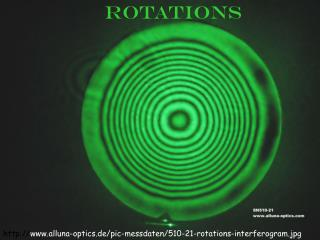# Rotations - PowerPoint PPT PresentationDownload PresentationRotations

RotationsDownload Presentation## Rotations

- - - - - - - - - - - - - - - - - - - - - - - - - - - E N D - - - - - - - - - - - - - - - - - - - - - - - - - - -
##### Presentation Transcript

1. Rotations http://www.alluna-optics.de/pic-messdaten/510-21-rotations-interferogram.jpg

2. Rotations • An image can be rotated about a fixed point. • The blades of a fan rotate about a fixed point. • An image can be rotated over two intersecting lines by using composite reflections. A C m B n Image A reflects over line m to B,image B reflects over line n to C. Image C is a rotation of image A. (modified HCPS)

3. B A 25 ° Angles of Rotation • In a given rotation, where A is the figure and A’ is the resulting figure after rotation, and X is the center of the rotation, the measure of the angle of rotation AXA’ is twice the measure of the angle formed by the intersecting lines of reflection. • Example: Given segment AB to be reflected over lines l and m, which intersect to form a 25° angle. Find the rotation image segment A’B’. (Solution is on the next slide.) l m X (modified HCPS)

4. Solution • Since the angle formed by the lines is 25°, the angle of rotation is 50°. • 1. Draw AXA’ so that its measure is 50° and AX = XA’. • 2. Draw BXB’ to measure 50° and BX = XB’. • 3. Connect A’ to B’ to form the rotation image of segment AB. B A’ B’ A 25 ° X

5. Four Definitions • A rotation is a transformation in which a figure is turned about a fixed point. A rotation is an isometry. • The fixed point of a rotation is called the center of rotation. • Rays drawn from the center of rotation to a point and its image form an angle called the angle of rotation. • A figure in the plane has rotational symmetry if the figure can be mapped onto itself by a clockwise rotation of 180° or less.

6. Rotations in a Coordinate Plane In a coordinate plane sketch the triangle with vertices (-1, 5), (3, 2), and (4, 5). Then rotate the triangle 90° clockwise about the origin, label your image. Then name the coordinates of the image. Coordinates

7. Generalize the Last Rotation If you look at the coordinates of the vertices of both the preimage and the image, the results can be summarized as follows: Note: The rotations can be done using patty paper if you are having difficulty. Trace the original figure (preimage) and the x and y axes. Rotate your copy the given degrees in the appropriate direction keeping the origin matched up.

8. A Second Example Rotate the triangle 180° clockwise about the origin. Label your image. Would your image be any different if the rotation were counterclockwise? NO

9. Look at Slopes The slopes of the two segments that are the same color (form a right angle and thus are perpendicular) are negative reciprocals of one another. This knowledge can help you do the rotation. The slopes of the different colored segments remain constant. Count the slope from A to the origin and repeat this count from the origin to A’. This knowledge can help you do the rotation.

10. A Third Example Rotate the triangle 90° counterclockwise about the origin. Label your image.

11. Rotational Symmetry Recall: A figure in the plane has rotational symmetry if the figure can be mapped onto itself by a clockwise rotation of 180° or less about its center. Determine whether the figure has rotational symmetry. If it does describe the rotational symmetry. Rotational symmetry of 90° No rotational symmetry Rotational symmetry of 180° Note: You could trace the figure onto patty paper and turn the copy around on top of the original and determine how many degrees it takes for the figures to match up.

12. Solution One Last Example Determine whether the figure has rotational symmetry. If it does describe the rotational symmetry. You could also consider the number of rotations in 90°. There are 2 of them so the rotational symmetry is 45°.